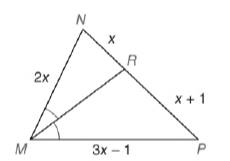Chapter 5.6, Problem 26EElementary Geometry For College St...

7th Edition
Alexander + 2 others
ISBN: 9781337614085

Solutions

Chapter
SectionElementary Geometry For College St...

7th Edition
Alexander + 2 others
ISBN: 9781337614085
Textbook Problem

Given: M R → bisects ∠ N M P , M N = 2 x , N R = x , R P = x + 1 , and M P = 3 x − 1Find: x

To determine

To find:

The value of x.

Explanation

Given:

MR bisects NMP, MN=2x,NR=x,RP=x+1,MP=3x1.

Theorem used:

Angle bisector theorem:

If a ray bisects one angle of a triangle, then it divides the opposite side into segments whose lengths are proportional to the lengths of the two sides that form the bisected angle.

Calculation:

We have MR bisects NMP.

By angle bisector theorem, we get

MNMP=RNRP.

Given that MN=2x,NR=x,RP=x+1,MP=3x1

Thus 2x3x1=xx+1

Still sussing out bartleby?

Check out a sample textbook solution.

See a sample solution

The Solution to Your Study Problems

Bartleby provides explanations to thousands of textbook problems written by our experts, many with advanced degrees!

Get Started

Simplify: 2436

Elementary Technical Mathematics

In Exercises 7-28, perform the indicated operations and simplify each expression. 10. 3x24xy4y2x2y(2yx)2x3y

Applied Calculus for the Managerial, Life, and Social Sciences: A Brief Approach

Perform the indicated operation for the following.

Contemporary Mathematics for Business & Consumers

Let A=B=C= Find a AB, b (A+CT)B, and c CTBABT.

Finite Mathematics for the Managerial, Life, and Social Sciences

Find for x = 3t2 + 1, y = t6 + 6t5. t4 + 5t3 4t3 + 15t2

Study Guide for Stewart's Multivariable Calculus, 8th